# (1 point) Suppose that the matrix A has the following eigenvalues and eigenvectors 2-2i and -2+2i Write the solution to...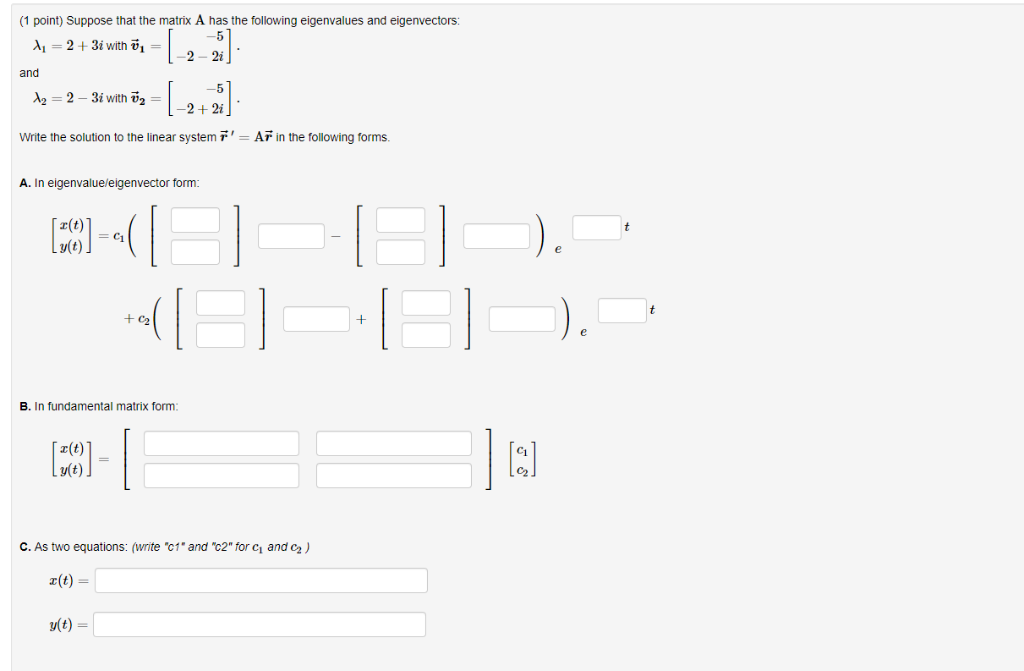(1 point) Suppose that the matrix A has the following eigenvalues and eigenvectors 2-2i and -2+2i Write the solution to the linear system AF in the following forms A. In eigenvalueleigenvector form r(t) B. In fundamental matrix form z(t) v(t) C. As two equations: (write "c1* and "c2" for ci and C2) a(t)- v(t)-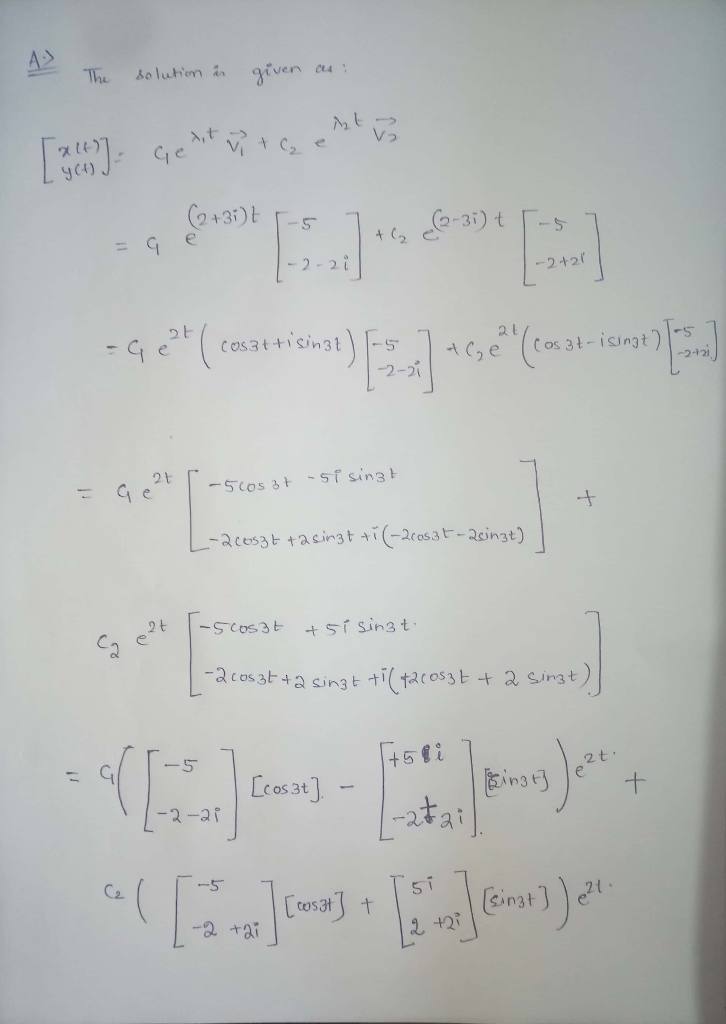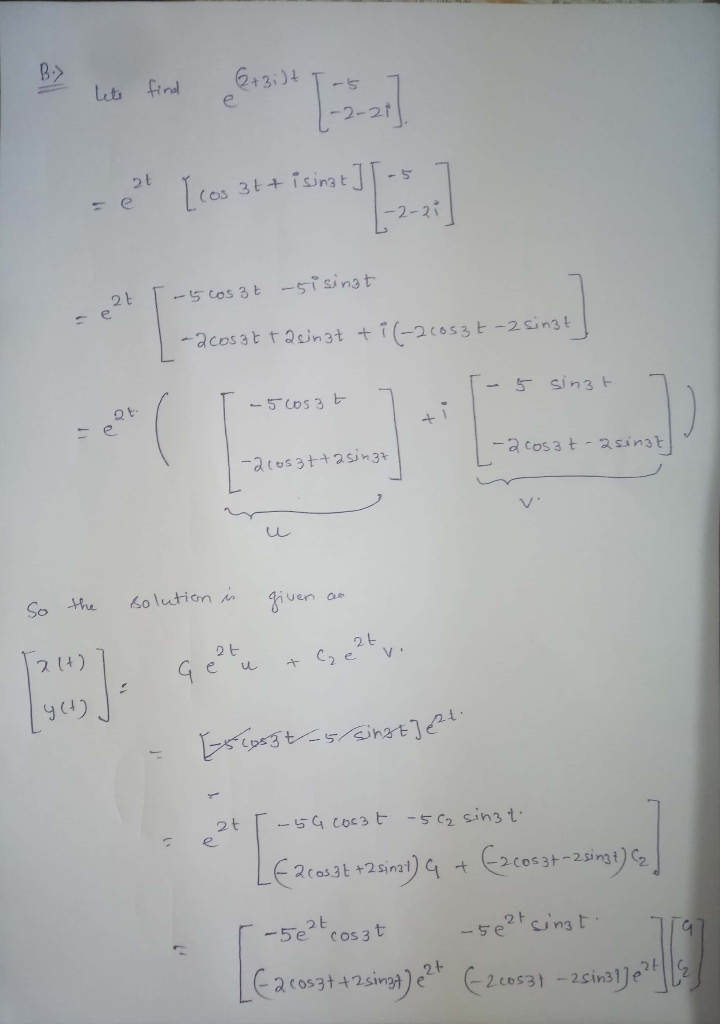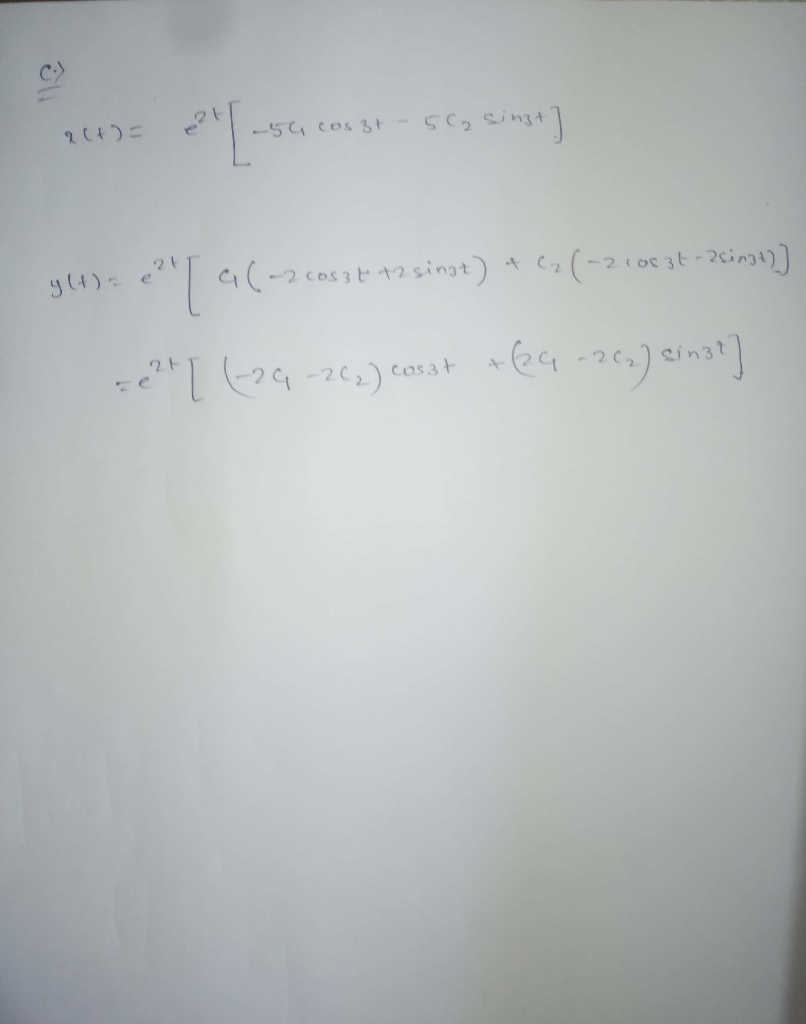##### Add Answer of: (1 point) Suppose that the matrix A has the following eigenvalues and eigenvectors 2-2i and -2+2i Write the solution to...
Similar Homework Help Questions
• ### Suppose that the matrix A A has the following eigenvalues and eigenvectors: (1 point) Suppose that...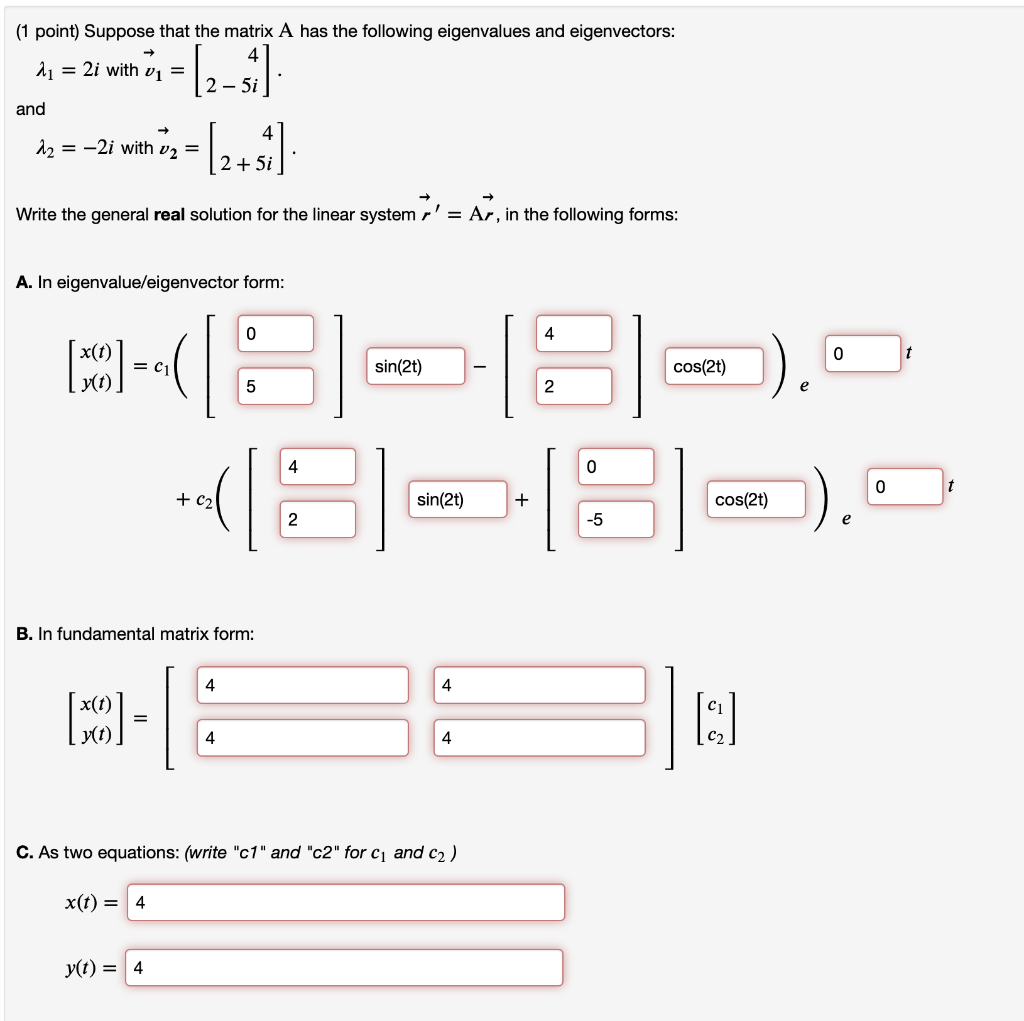Suppose that the matrix A A has the following eigenvalues and eigenvectors: (1 point) Suppose that the matrix A has the following eigenvalues and eigenvectors: 2 = 2i with v1 = 2 - 5i and - 12 = -2i with v2 = (2+1) 2 + 5i Write the general real solution for the linear system r' = Ar, in the following forms: A. In eigenvalue/eigenvector form: 0 4 0 t MODE = C1 sin(2t) cos(2) 5 2 4 0 0...

• ### (1 point) Suppose that the matrix A has the following eigenvalues and eigenvectors: 4 = 2...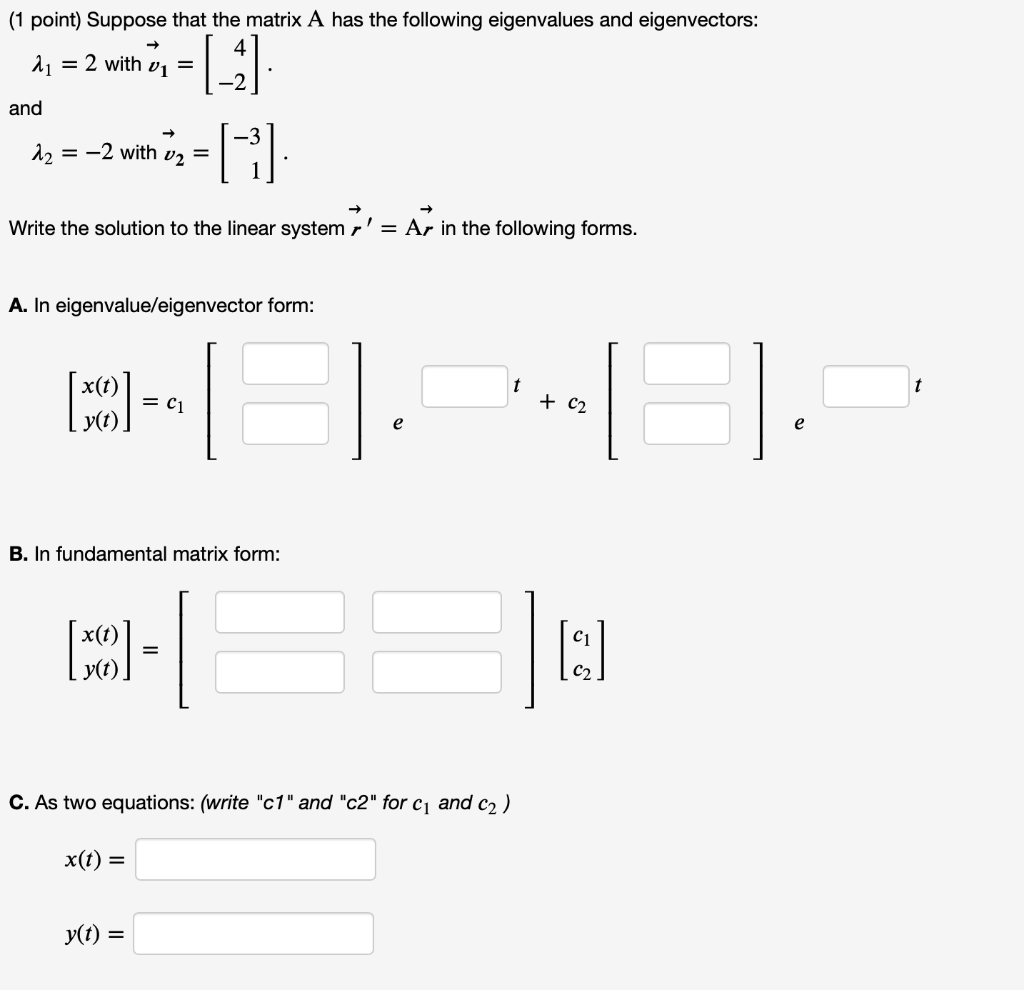(1 point) Suppose that the matrix A has the following eigenvalues and eigenvectors: 4 = 2 with vi = and |_ G 12 = -2 with v2 = Write the solution to the linear system r' = Ar in the following forms. A. In eigenvalue/eigenvector form: x(t) (50) = C1 + C2 e e B. In fundamental matrix form: (MCO) = I: C. As two equations: (write "c1" and "c2" for C1 and c2) x(t) = yt) =

• ### (1 point) Suppose that the matrix A has the following eigenvalues and eigenvectors: A1 = 4...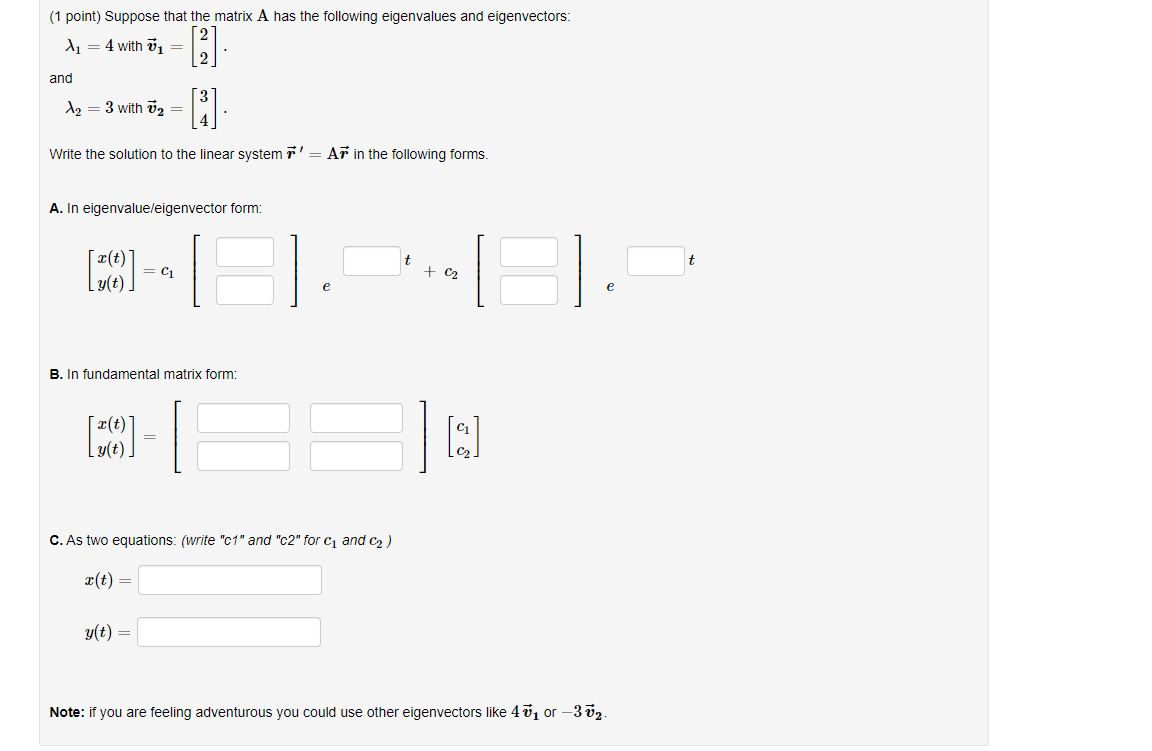(1 point) Suppose that the matrix A has the following eigenvalues and eigenvectors: A1 = 4 with = and  [i] Az = 3 with Ū2 = Write the solution to the linear system r' = Ar in the following forms. A. In eigenvalue/eigenvector form: t (10) -- + C2 e e B. In fundamental matrix form: (39) - g(t). C. As two equations: (write "c1" and "c2" for C and C2) X(t) = g(t) = Note: if you are...

• ### (1 point) Suppose that the matrix A has repeated eigenvalue with the following eigenvector and generalized...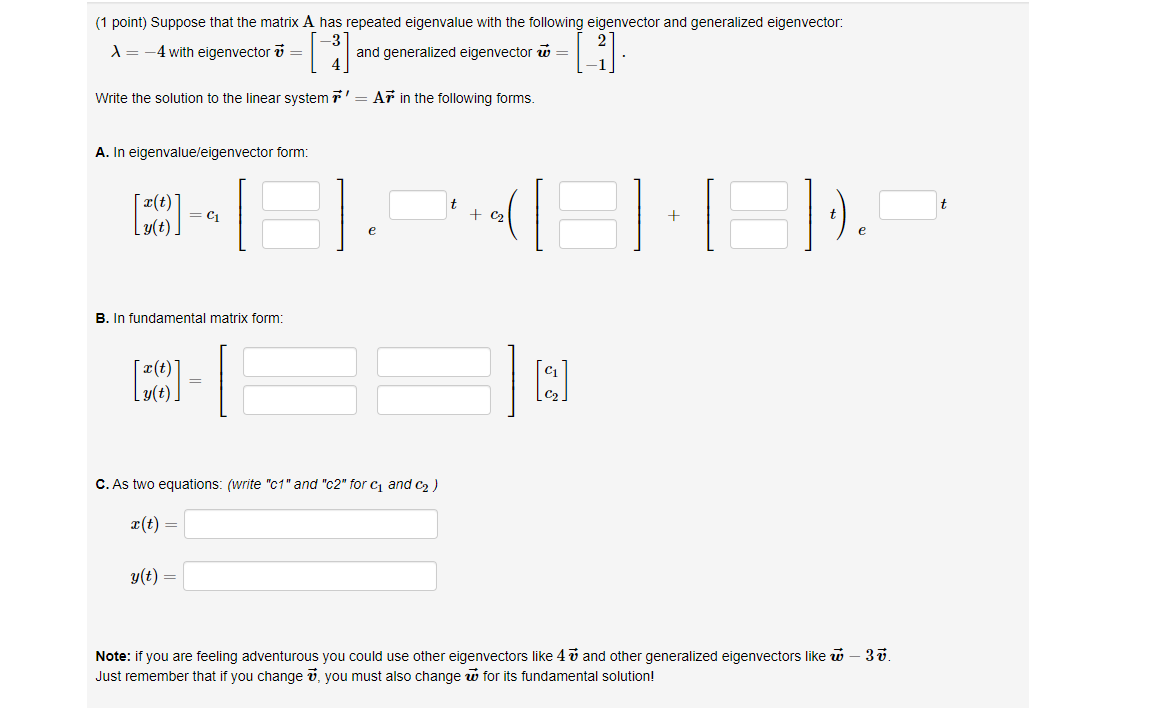(1 point) Suppose that the matrix A has repeated eigenvalue with the following eigenvector and generalized eigenvector: X= -4 with eigenvector v = and generalized eigenvector ū= [] (-1) Write the solution to the linear system r' = Ar in the following forms. A. In eigenvalue/eigenvector form: t t [CO] = C1 + C2 + I g(t). e . - 1 B. In fundamental matrix form: [CO] C. As two equations: (write "c1" and "c2" for 1 and 2) X(t)...

• ### (1 point) Suppose that the matrix A has repeated eigenvalue with the following eigenvector and generalized...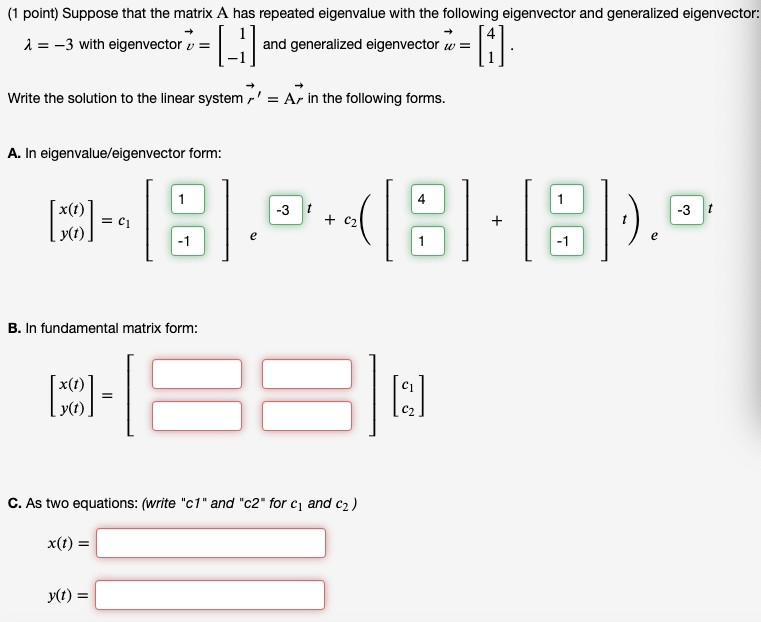(1 point) Suppose that the matrix A has repeated eigenvalue with the following eigenvector and generalized eigenvector: i = -3 with eigenvector v = and generalized eigenvector w= = [-] =  Write the solution to the linear system r' = Ar in the following forms. A. In eigenvalue/eigenvector form: 1 (O) = - 18.05.8:8)... y(t) B. In fundamental matrix form: (O)- x(1) y(t) [:] C. As two equations: (write "c1" and "c2" for c and c2) X(t) = yt)...

• ### (1 point) Consider the linear system "(-1: 1) y. a. Find the eigenvalues and eigenvectors for...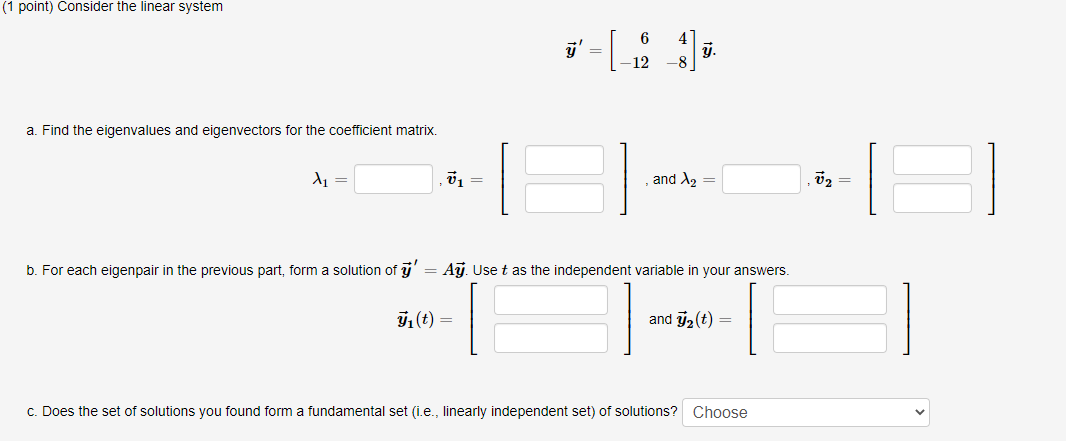(1 point) Consider the linear system "(-1: 1) y. a. Find the eigenvalues and eigenvectors for the coefficient matrix. 1 v1 = and 2 V2 b. For each eigenpair in the previous part, form a solution of y' = Ay. Use t as the independent variable in your answers. (t) = and yz(t) c. Does the set of solutions you found form a fundamental set (i.e., linearly independent set) of solutions? Choose

• ### Matrix Question!eigenvectors and eigenvalues for symmetric matrix?

Suppose A is a symmetric N X N matrix with eigenvectors vi, i = 1; 2; 3 ...N withcorresponding eigenvalues ?i, i = 1; 2; 3 ...N.Pick any two distinct eigenvalues (assuming such a pair exists). Let's call them ?1 and?2 and their corresponding eigenvectors v1 and v2.Write down the matrix equations that show that v1 and v2 are eigenvectors of A.

• ### 8. 20 pts.] Suppose that a 2 x2 matrix A has the following eigenvalues and eigenvectors:...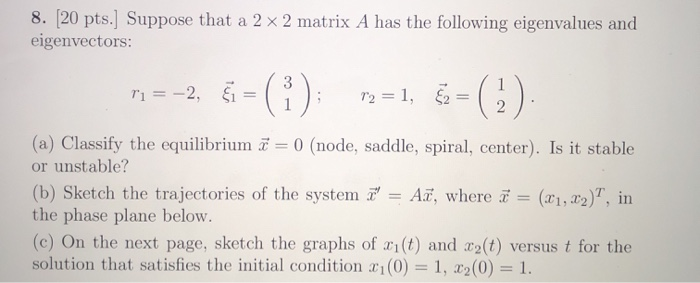8. 20 pts.] Suppose that a 2 x2 matrix A has the following eigenvalues and eigenvectors: () 12, 1 r2=1, 2 2 (a) Classify the equilibrium 0 (node, saddle, spiral, center). Is it stable or unstable? (b) Sketch the trajectories of the system A , where a the phase plane below. (c) On the next page, sketch the graphs of r1 (t) and 2(t) versus t for the solution that satisfies the initial condition x1(0) = 1, x2(0) = 1...

• ### (1 point) In this problem you will solve the nonhomogeneous system 1 A. Write a fundamental...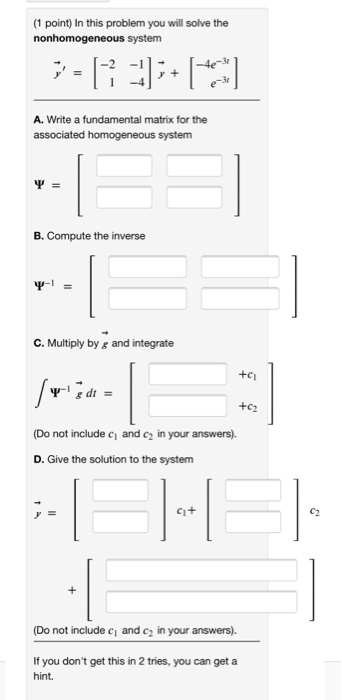(1 point) In this problem you will solve the nonhomogeneous system 1 A. Write a fundamental matrix for the associated homogeneous system = B. Compute the inverse C. Multiply by g and integrate +ci dt +c2 (Do not include c and c2 in your answers). D. Give the solution to the system C1+ (Do not include ci and c2 in your answers). If you don't get this in 2 tries, you can get a hint. (1 point) In this problem...

• ### Solving 1. Let -Ar, A constant, with real and distinct eigenvalues 3-1 0 2-2 3 A...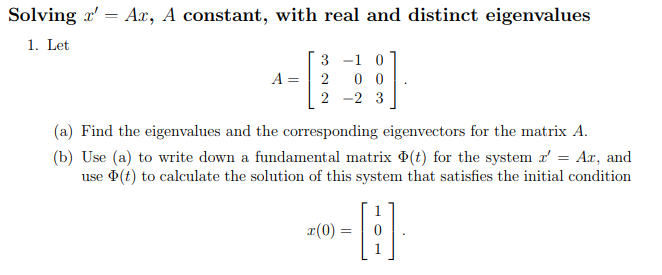Solving 1. Let -Ar, A constant, with real and distinct eigenvalues 3-1 0 2-2 3 A 2 0 0 (a) Find the eigenvalues and the corresponding eigenvectors for the matrix A. (b) Use (a) to write down a fundamental matrix Φ(t) for the system z' = Az, and use Φ(t) to calculate the solution of this system that satisfies the initial condition (0)0

Free Homework App